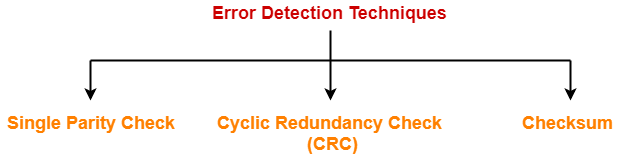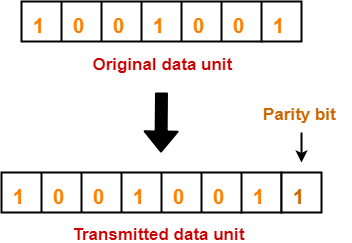# Error Detection in Computer Networks | Parity Check

## Error Detection in Computer Networks-

When sender transmits data to the receiver, the data might get scrambled by noise or data might get corrupted during the transmission.

 Error detection is a technique that is used to check if any error occurred in the data during the transmission.

## Error Detection Methods-

Some popular error detection methods are-1. Single Parity Check
2. Cyclic Redundancy Check (CRC)
3. Checksum

## Single Parity Check-

In this technique,

• One extra bit called as parity bit is sent along with the original data bits.
• Parity bit helps to check if any error occurred in the data during the transmission.

## Steps Involved-

Error detection using single parity check involves the following steps-

## Step-01:

At sender side,

• Total number of 1’s in the data unit to be transmitted is counted.
• The total number of 1’s in the data unit is made even in case of even parity.
• The total number of 1’s in the data unit is made odd in case of odd parity.
• This is done by adding an extra bit called as parity bit.

## Step-02:

• The newly formed code word (Original data + parity bit) is transmitted to the receiver.

## Step-03:

• The total number of 1’s in the received code word is counted.

Then, following cases are possible-

• If total number of 1’s is even and even parity is used, then receiver assumes that no error occurred.
• If total number of 1’s is even and odd parity is used, then receiver assumes that error occurred.
• If total number of 1’s is odd and odd parity is used, then receiver assumes that no error occurred.
• If total number of 1’s is odd and even parity is used, then receiver assumes that error occurred.

## Parity Check Example-

Consider the data unit to be transmitted is 1001001 and even parity is used.

Then,

### At Sender Side-

• Total number of 1’s in the data unit is counted.
• Total number of 1’s in the data unit = 3.
• Clearly, even parity is used and total number of 1’s is odd.
• So, parity bit = 1 is added to the data unit to make total number of 1’s even.
• Then, the code word 10010011 is transmitted to the receiver.• After receiving the code word, total number of 1’s in the code word is counted.
• Even parity is used and total number of 1’s is even.
• So, receiver assumes that no error occurred in the data during the transmission.

• This technique is guaranteed to detect an odd number of bit errors (one, three, five and so on).
• If odd number of bits flip during transmission, then receiver can detect by counting the number of 1’s.

## Limitation-

• This technique can not detect an even number of bit errors (two, four, six and so on).
• If even number of bits flip during transmission, then receiver can not catch the error.

### EXAMPLE

• Consider the data unit to be transmitted is 10010001 and even parity is used.
• Then, code word transmitted to the receiver = 100100011
• Consider during transmission, code word modifies as 101100111. (2 bits flip)
• On receiving the modified code word, receiver finds the number of 1’s is even and even parity is used.
• So, receiver assumes that no error occurred in the data during transmission though the data is corrupted.

To gain better understanding about single parity check,

Watch this Video Lecture

Next Article- Cyclic Redundancy Check

Get more notes and other study material of Computer Networks.

Watch video lectures by visiting our YouTube channel LearnVidFun.

SummaryArticle Name
Error Detection in Computer Networks | Parity Check
Description
Error Detection in Computer Networks is a method to detect errors in the data introduced during transmission. Parity Check uses a parity bit to perform error detection. Cyclic Redundancy Check (CRC) and Checksum are other error detection methods.
Author
Publisher Name
Gate Vidyalay
Publisher Logo